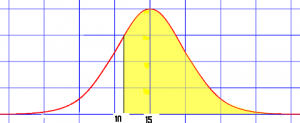# Calculus Based Statistics

Contents:

## What is Calculus Based Statistics?

Calculus Based statistics takes the four core concepts of calculus (Continuity, Limits, Definite integral, Derivative) and applies them to statistical theory. Essentially, non-calculus based statistics is for consumers of statistics and calculus based statistics is more suited for people who want to create statistics (Columbia, 2021).

## Elementary Statistics vs. Calculus Based Statistics

There are many similarities and some important differences between Elementary Statistics and Calculus Based Statistics. Whichever class you take, you’re going to cover the same core concepts including ANOVA, Confidence intervals, Correlation, Regression and Statistical Inference.

The main difference is in how these topics are approached. For example, in a basic stats class, confidence intervals are introduced as a way to describe the distribution of parameters (i.e. how spread out your estimated results are). The focus is on how to make and interpret these intervals. With a calculus based statistics approach, functions are derived for these intervals. In a non-calc class, you might study the survival function for a certain species. In a calculus based course, you might take that same survival function and integrate it to show that the average lifetime for that species is the area under the curve.

In non-calculus statistics classes the focus is on using statistics. Calculus based statistics is more about creating the statistics (for others to consume). It is generally a more rigorous class that will help you to:

• Create statistics from scratch for any data type,
• Understand where many statistical rules and assumptions come from,
• Extend basic tests and procedures to non-standard situations.

## Calculus Based Statistics: ExamplesThe normal distribution is one example of a continuous function that can be explored with calculus.
Some areas of statistics are difficult or impossible to evaluate without calculus.
The following table lists some examples of where you could (or must) apply calculus:

## Typical Contents for Calculus Based Statistics

Many of the following concepts are found in a calculus based statistics class. They are either not covered at all in an elementary statistics class or are skimmed over:

On the other hand, you probably won’t see these advanced calculus concepts in a calculus based statistics class or an elementary statistics class. In fact, you probably won’t come across them at all unless you dive into the realm of mathematical statistics:

## Should I Take Calculus or Non-Calculus Based Statistics?

Which course you take largely depends on what your future goals are.

Few disciplines really need calculus based statistics. Those that do include economics, mathematical statistics and many research-heavy fields. In addition, some academic disciplines encourage (or require) a calculus background in addition to statistics. For example, statistics and calculus are highly sought after skills by research-active life scientists at the University of Arizona (Watkins, 2010).

As elementary statistics focuses more on data analysis, it’s well-suited to pre-med students, social science majors, and business majors.

For other disciplines, it’s a grey area. For example, data scientists can get away with not knowing calculus for many positions but many career opportunities will require you to use calculus to explore data.

## Definitions and How To Articles

The following how to articles cover the basics of calculus: the tools you will likely need to get you through an introductory calculus based statistics class.

## Calculus based Statistics: Definitions

These full definitions are some of the topics you may come across in a calculus based statistics class:

## Calculus based Statistics: References

Columbia University. (2021). Statistics. Retrieved January 4, 2021 from: http://bulletin.columbia.edu/columbia-college/departments-instruction/statistics/
DeGroot, M. & Schervish, J. (2019). Probability and Statistics (Classic Version), 4th edition.
Pearson.
Gemignani, M. (2004). Calculus and Statistics. Dover.
Watkins, J. (2010). On a Calculus based Statistics Course for Life Science Students. CBE Life Sci Educ. 2010 Fall; 9(3): 298–310

CITE THIS AS:
Stephanie Glen. "Calculus Based Statistics" From StatisticsHowTo.com: Elementary Statistics for the rest of us! https://www.statisticshowto.com/probability-and-statistics/calculus-based-statistics/
---------------------------------------------------------------------------Need help with a homework or test question? With Chegg Study, you can get step-by-step solutions to your questions from an expert in the field. Your first 30 minutes with a Chegg tutor is free!